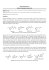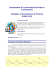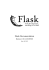# Ultimate Gas Laws Worksheet

## Transcription

Ultimate Gas Laws Worksheet
```Ultimate Gas Laws Worksheet
Useful stuff:
Name_______________________________________________
PV = nRT
R=
Pt = P1 + P2 + P3 . . .
=
=
Pay attention to units!!
1. A fixed quantity of gas at 21°C has a pressure of 752 Torr and occupies a volume of 5.12 L
a) Calculate the volume the gas will occupy if the pressure is increased to 1.88 atm while the
temperature is held constant.
b) Calculate the volume the gas will occupy if the temperature is increased to 175°C while the
pressure is held constant.
2. An aerosol spray can with a volume of 250mL contains 2.30 g of propane gas (C3H8) as a propellant.
a) If the can is at 23°C, what is the pressure in the can?
b) What volume would the propane occupy at STP?
c) The can's label says that exposure to temperatures above 130°C may cause the can to burst. What is
the pressure in the can at this temperature?
3. Calcium hydride (CaH2) reacts with water to form hydrogen gas:
CaH2(s) + 2 H2O(l) → Ca(OH)2(aq) + 2 H2(g)
The reaction is sometimes used to inflate life rafts, weather balloons, and the like, when a simple, compact
means of generating H2 is needed. How many grams of CaH2 are needed to generate 145L of H2 gas if the
pressure is 825 Torr at 21°C
4. Acetylene gas (C2H2) can be prepared by the reaction of calcium carbide with water.
CaC2(s) + 2 H2O(l) → Ca(OH)2(s) + C2H2(g)
Calculate the volume of C2H2 that is collected over water at 23.0°C by reaction of 0.1580 g of CaC2 if the total
pressure of the gas is 766 torr? (You can get vapor pressure of water from the chart posted in the discussion
conference).
5. Calculate the density of sulfur hexafluoride gas at 93.1kPa and 21°C
6. Calculate the molar mass of a gas that has a density of 7.135g/L at 12°C and 0.993 atm.
7. A diver uses a gas cylinder with a volume of 10.0L and a content of 51.2g of O2 and 32.6 g of He. Calculate the
partial pressure of each gas and the total pressure if the temperature of the gas is 19°C.
8. In a mixture of 15.08 g of O2, 8.17 g of N2, and 2.64g of H2
a) What are the mole fractions of each component?
b) What is the partial pressure in atm of each component of this mixture if it is contained in a 15.50 L
9. A 25.0 L tank is filled with He gas at a pressure of 100. atm and at 25°C. How many balloons (each holds 2.00
L) can be inflated to a pressure of 1.00 atm, assuming that the temperature remains constant and that the tank
cannot be emptied below 1.00 atm.
10. A student collected a sample of hydrogen gas by the displacement of water as shown by the diagram above.
The relevant data are given in the following table.
GAS SAMPLE DATA
Volume of sample
90.0 mL
Temperature
25°C
Atmospheric Pressure
745 mm Hg
Equilibrium Vapor Pressure of 23.8 mm Hg
H2O (25°C)
a)Calculate the number of moles of hydrogen gas collected.
b) Calculate the number of molecules of water vapor in the sample of gas.
c) Calculate the ratio of the rate the hydrogen molecules would escape from a hole in the tube to the
rate or the water vapor molecules in the sample.
d) Which of the two gases, H2 or H2O, deviates more from ideal behavior? Explain your answer.
11. A glass 5.00 L flask contains 0.176 mol of NO(g) at 298 K. A 0.176 mol sample of O2(g) is added to the flask,
where a reaction occurs to produce NO2(g).
a)Write the balanced equation for the reaction.
b) Calculate the total pressure, in atm, in the cylinder at 298 K after the reaction is complete.
(Note this one is tricky - you have to determine the limiting reagent and figure out how many moles of gas are
formed and how many moles of excess reagent are left over in order to get your total number of moles)
12. A sugar cube has a mass of 25.5 g (sucrose has a formula of C12H22O11). Digesting sucrose involves a
combustion reaction. What total volume of gas (CO2 and H2O) would be produced from this reaction if it takes
place at 23.0°C and 1.2 atm?
13. Three 1.00 L flasks at 25.0 °C and 101.3 kPa pressure contain: CH4 (flask A), CO2 (flask B) and NH3 (flask C).
Which flask (or none) contains 0.041 mol of gas?
14. 20.0 g each of helium and an unknown diatomic gas are combined in a 1500. mL container. If the
temperature is 298 K, and the pressure inside is 86.11 atm, what is the unknown gas?
15. A sample of gas (1.90 mol) is in a flask at 21.0 °C and 697.0 mm Hg. The flask is now opened and more gas is
added to the flask. The new pressure is 795.0 mm Hg and the temperature is now 26.0 °C. How many moles of
gas are now in the flask?
```

### 1. Define spontaneous generation. The idea that a mysterious life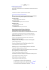### June 2013 - Goodsoil Credit Union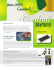### Problem Set 1 – October 2014 ion is: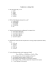### Practice problems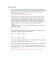### 1- Calculate the percent by mass of potassium... 295 mL of water. Density of water is 0.997 g/mL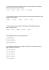### CML 100 Tutorial 3 SS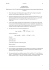### Suggested problems Chapter 19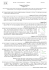### Version A Section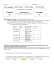### 251. The Fleaker - UC DRC Home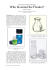### Louise Irvine, the renowned Royal Doulton expert and author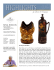### Chemical Kinetics AP Problems Quiz Numbers 1 to6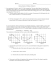### Problem Set Seven Solution Key: Version B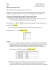### 2015 Rules and Information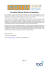### Review packet Answer key - Rejman Chemistry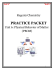### Preparatory problems 28 and 29, corrections (PDF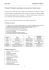### 3. group 2 and group 7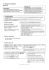### Reaction 1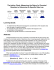### Wellbutrin/Zyban synthesis